11 篇文章 0 订阅

决策树

martin

基本概念

1. 当前节点包含的样本全部属于同一个类别，无需划分。
2. 当前属性集为空，或是所有样本在所有属性集上取值相同，无法划分。此时，把当前节点标记为叶节点，并将其类别设定为该节点所含样本最多的类别。
3. 当前节点包含的样本集合为空，不能划分。此时，同样把当前节点标记为叶节点，但将其类别设定为其父节点所含样本最多的类别。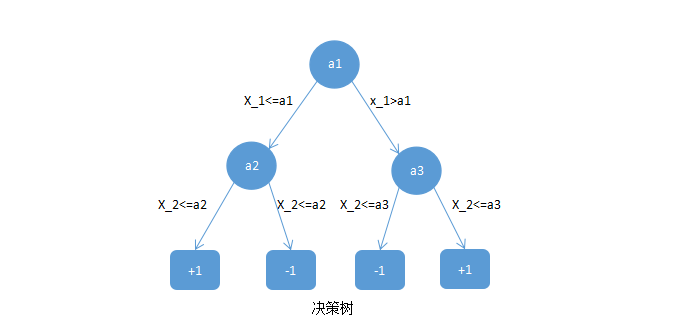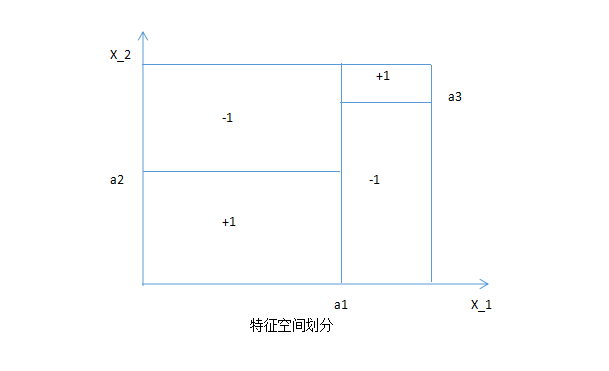ID3

E(D)=k=1ypklog2pk

aGain(D,a)=E(D)v=1v|Dv||D|E(Dv)

1青绿蜷缩浊响清晰凹陷硬滑
2乌黑蜷缩沉闷清晰凹陷硬滑
3乌黑蜷缩浊响清晰凹陷硬滑
4青绿蜷缩沉闷清晰凹陷硬滑
5浅白蜷缩浊响清晰凹陷硬滑
6青绿稍蜷浊响清晰稍凹软粘
7乌黑稍蜷浊响稍糊稍凹软粘
8乌黑稍蜷浊响清晰稍凹硬滑
9乌黑稍蜷沉闷稍糊稍凹硬滑
10青绿硬挺清脆清晰平坦软粘
11浅白硬挺清脆模糊平坦硬滑
12浅白蜷缩浊响模糊平坦软粘
13青绿稍蜷浊响稍糊凹陷硬滑
14浅白稍蜷沉闷稍糊凹陷硬滑
15乌黑稍蜷浊响清晰稍凹软粘
16浅白蜷缩浊响模糊平坦硬滑
17青绿蜷缩沉闷稍糊稍凹硬滑

E(D)=k=12pklog2pk=(817log2817+917log2917)=0.998

D1(=绿) $D^1(色泽=青绿)$，包含编号为 {1,4,6,10,13,17} $\{1,4,6,10,13,17\}$6个样例，于是 p1=36,p2=36 $p_1={3\over 6},p_2={3\over 6}$
D2(=) $D^2(色泽=乌黑)$，包含编号为 {2,3,7,8,9,15} $\{2,3,7,8,9,15\}$6个样例，于是 p1=46,p2=26 $p_1={4\over 6},p_2={2\over 6}$
D3(=) $D^3(色泽=浅白)$，包含编号为 {5,11,12,14,16} $\{5,11,12,14,16\}$5个样例，于是 p1=15,p2=45 $p_1={1\over 5},p_2={4\over 5}$

E(D1)=36log2(36)36log2(36)=1.000

E(D2)=46log2(46)26log2(26)=0.918

E(D3)=15log2(15)45log2(45)=0.722

Gain(D,)=E(D)v=13|Dv||D|E(Dv)=0.998(617×1.000+617×0.918+517×0.722)=0.109

Gain(D,)=0.143

Gain(D,)=0.141

Gain(D,)=0.381

Gain(D,)=0.289

Gain(D,)=0.006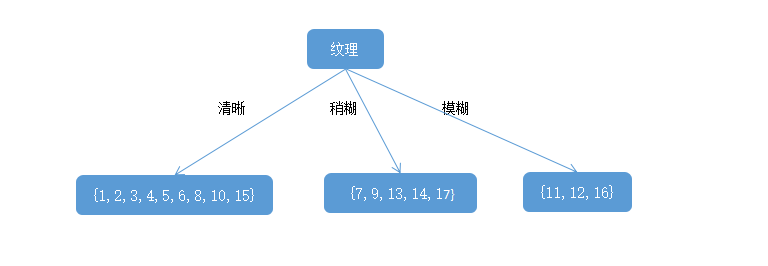E(D1)=k=12pklog2pk=(79log279+29log229)=0.764

D1(=绿) $D^1(色泽=青绿)$，包含编号为 {1,4,6,10} $\{1,4,6,10\}$4个样例，于是 p1=34,p2=14 $p_1={3\over 4},p_2={1\over 4}$
D2(=) $D^2(色泽=乌黑)$，包含编号为 {2,3,8,15} $\{2,3,8,15\}$4个样例，于是 p1=34,p2=14 $p_1={3\over 4},p_2={1\over 4}$
D3(=) $D^3(色泽=浅白)$，包含编号为 {5} $\{5\}$1个样例，于是 p1=11,p2=01=0 $p_1={1\over 1},p_2={0\over 1}=0$

E(D1)=34log2(34)14log2(14)=0.811

E(D2)=34log2(34)14log2(14)=0.811

E(D3)=11log2(11)0×log2(0)=0

Gain(D1,)=E(D1)v=13|Dv||D|E(Dv)=0.764(49×0.811+49×0.811+0)=0.043

Gain(D1,)=0.458

Gain(D,)=0.331

Gain(D,)=0.458

Gain(D,)=0.458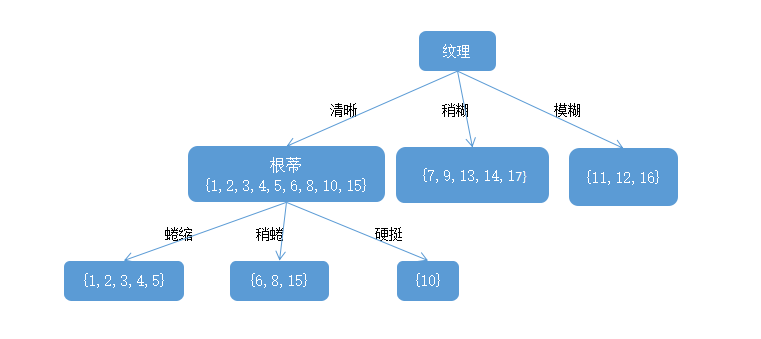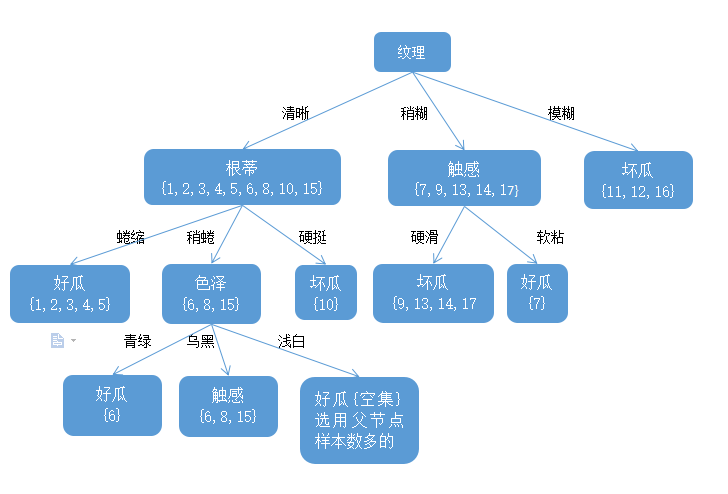C4.5

Gainraio(D,a)=Gain(D,a)IV(a)

IV(a)=v=1v|Dv||D|log2|Dv||D|

IV(a)称为属性 a $a$的“固有值”。属性a$a$的可能值数目越多(即V越大)，则IV(a)的值通常会越大。例如，对于上表的西瓜数据集，有：
IV()=1217log21217517log2517=0.874(v=2)

IV()=617log2617617log2617517log2517=1.580(v=3)

IV()==4.088(v=17)

CART

CART决策树是一棵二叉树，内部节点特征取值为“是”和“否”，左分支是取值为“是”的分支，右分支是取值为“否”的分支。CART决策树使用“基尼系数”来选择划分属性。基尼系数的定义如下：

Gini(D)=k=1ypk(1pk)=1k=1kp2k

Gini(D,a)=v=1v|Dv||D|Gini(Dv)

1 青年 一般
2 青年
3 青年
4 青年 一般
5 青年 一般
6 中年 一般
7 中年
8 中年
9 中年 非常好
10 中年 非常好
11 老年 非常好
12 老年
13 老年
14 老年 非常好
15 老年 一般

Gini(D,)=515[1(252+352)]+1015[1(7102+3102)]=0.44

Gini(D,)=515[1(352+252)]+1015[1(6102+4102)]=0.48

Gini(D,)=515[1(452+152)]+1015[1(5102+5102)]=0.44

Gini(D,)=515[1(552)]+1015[1(4102+6102)]=0.32

Gini(D,)=1015[1(4102+6102)]+515[1(552)]=0.32

Gini(D,)=615[1(662)]+915[1(392+692)]=0.27

Gini(D,)=915[1(392+692)]+615[1(662)]=0.27

Gini(D,)=0.36

Gini(D,)=0.47

Gini(D,)=0.32

Gini(D,非常好)最小，所以信贷非常好作为最优切分点。

剪枝处理

1青绿蜷缩浊响清晰凹陷硬滑
2乌黑蜷缩沉闷清晰凹陷硬滑
3乌黑蜷缩浊响清晰凹陷硬滑
6青绿稍蜷浊响清晰稍凹软粘
7乌黑稍蜷浊响稍糊稍凹软粘
10青绿硬挺清脆清晰平坦软粘
14浅白稍蜷沉闷稍糊凹陷硬滑
15乌黑稍蜷浊响清晰稍凹软粘
16浅白蜷缩浊响模糊平坦硬滑
17青绿蜷缩沉闷稍糊稍凹硬滑

4青绿蜷缩沉闷清晰凹陷硬滑
5浅白蜷缩浊响清晰凹陷硬滑
8乌黑稍蜷浊响清晰稍凹硬滑
9乌黑稍蜷沉闷稍糊稍凹硬滑
11浅白硬挺清脆模糊平坦硬滑
12浅白蜷缩浊响模糊平坦软粘
13青绿稍蜷浊响稍糊凹陷硬滑

前剪枝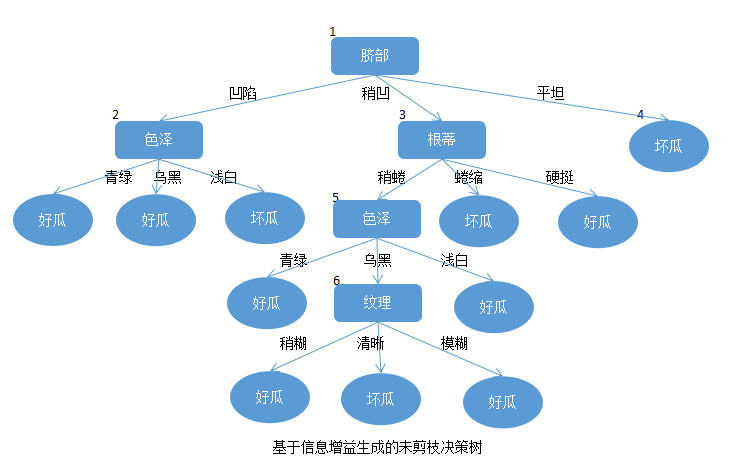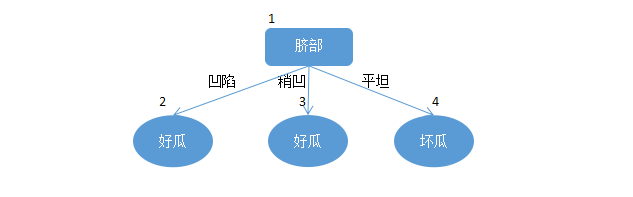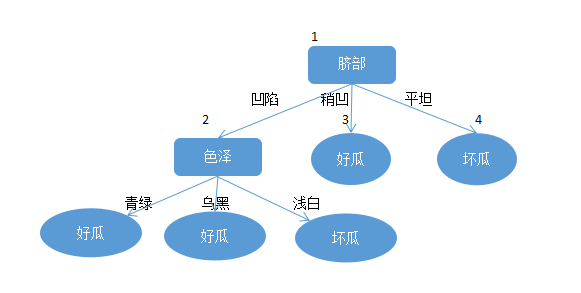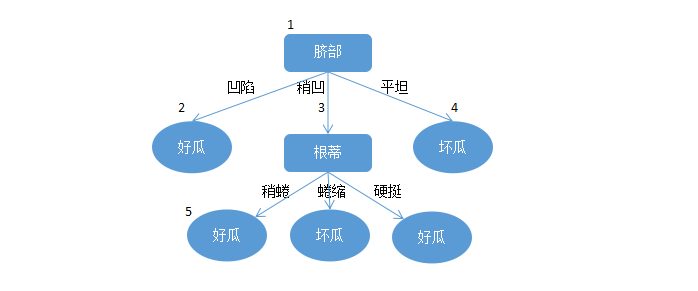后剪枝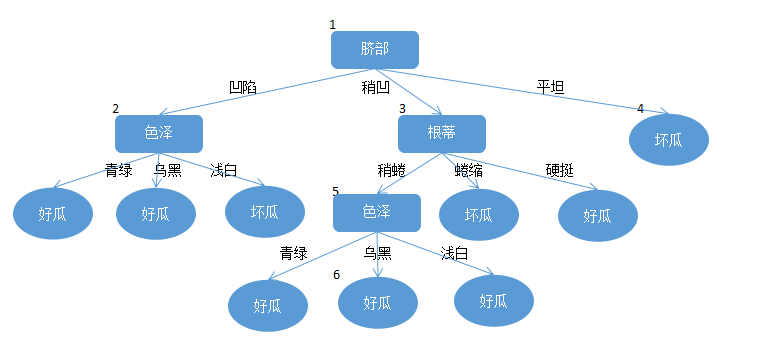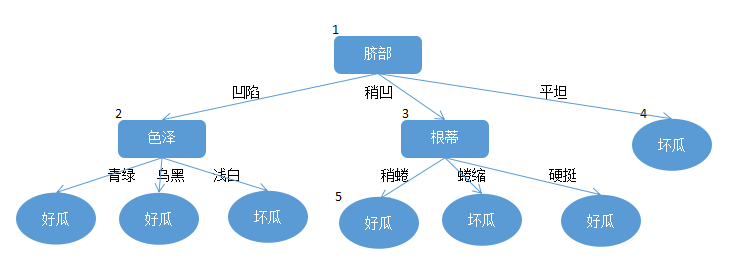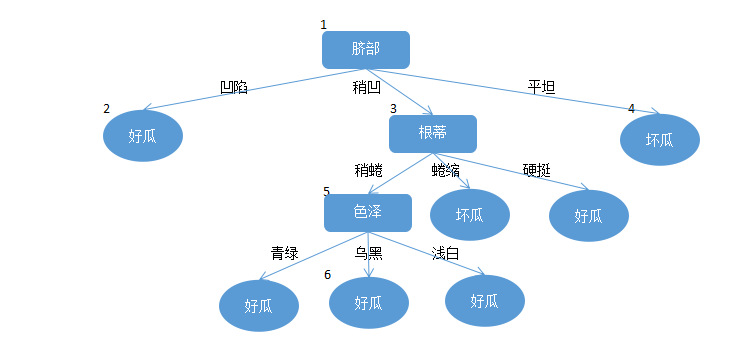《机器学习》周志华
《统计学习方法》李航

03-2040
08-234416

03-201万+
02-165820
12-234万+
07-026876
07-185万+
07-012058
06-142991
04-175872
08-23286
11-162278
06-02346
05-099147
09-08350
09-152488
05-061万+
09-202068
02-121万+ice_martin

¥2 ¥4 ¥6 ¥10 ¥20余额支付 (余额：-- )扫码支付获取中扫码支付点击重新获取扫码支付1.余额是钱包充值的虚拟货币，按照1:1的比例进行支付金额的抵扣。
2.余额无法直接购买下载，可以购买VIP、C币套餐、付费专栏及课程。余额充值